• 编译原理词法分析器实验报告含源代码，还有状态转换图。C语言实现
• 编译原理词法分析 要求：对如下工作进行展开描述 (1) 给出语言的词法规则描述 • 标识符、关键字、整常数、字符常数、浮点常数 • 单界符：＋，－，×，：，… • 双界符：/*，:=，… • 注释 (2) 针对这种单词的...
编译原理词法分析
要求：对如下工作进行展开描述
(1) 给出语言的词法规则描述
• 标识符、关键字、整常数、字符常数、浮点常数
• 单界符：＋，－，×，：，…
• 双界符：/*，:=，…
• 注释
(2) 针对这种单词的状态转换图和程序框图
(3) 核心数据结构的设计
如符号表、关键字等
(4) 错误处理
错误的位置及类型等
#include<iostream>
#include<fstream>
#include<cstdio>
#include<cstring>
#include<string>
#include<cstdlib>

using namespace std;

int aa;// fseek的时候用来接着的
string  word = "";
string  reserved_word;//保留
char buffer;//每次读进来的一个字符
int num = 0;//每个单词中当前字符的位置
int line = 1; //行数
int row = 1; //列数，就是每行的第几个
bool flag; //文件是否结束了
int flag2;//单词的类型

//设置保留字
void set_reserve()
{
reserved_word = "procedure";
reserved_word = "def";
reserved_word = "if";
reserved_word = "else";
reserved_word = "while";
reserved_word = "call";
reserved_word = "begin";
reserved_word = "end";
reserved_word = "and";
reserved_word = "or";

reserved_word = "return";
reserved_word = "float";
reserved_word = "double";
reserved_word = "void";
reserved_word = "cin";
reserved_word = "cout";
reserved_word = "char";
reserved_word = "for";
reserved_word = "true";
reserved_word = "false";
reserved_word = "int";
reserved_word = "bool";
reserved_word = "main";
reserved_word = "#";
reserved_word = "include";
}

//看这个字是不是字母
bool judge_word(char x)
{
if (x >= 'a' && x <= 'z' || x >= 'A' && x <= 'Z') {
return true;
}
else return false;
}

//看这个字是不是数字
bool judge_number(char x)
{
if (x >= '0' && x <= '9') {
return true;
}
else return false;
}

//看这个字符是不是界符
bool judge_jiefu(char x)
{
if (x == '(' || x == ')' || x == ',' || x == ';' || x == '{' || x == '}' || x == '"' || x == '\'') {
return true;
}
else return false;
}

//加减乘
bool judge_yunsuanfu1(char x)
{
if (x == '+' || x == '-' || x == '*')
{
return true;
}
else return false;
}

//等于 赋值，大于小于 大于等于，小于等于，大于小于
bool judge_yunsuannfu2(char x)
{
if (x == '=' || x == '>' || x == '<') {
return true;
}
else return false;
}

//这个最大的函数的总体作用是从文件里读一个单词
int scan(FILE* fp)
{
buffer = fgetc(fp);
if (feof(fp)) {
flag = 0; return 0;
}
//cout<<buffer;
else if (buffer == ' ')
{
row++;
return 0;
}
else if (buffer == '\n')
{
line++;
row = 1;
return 0;
}

//如果是字母开头或'_' 看关键字还是普通单词
else if (judge_word(buffer) || buffer == '_')
{
word += buffer; row++;
while ((buffer = fgetc(fp)) && (judge_word(buffer) || judge_number(buffer) || buffer == '_'))
{
word += buffer; row++;
}
if (feof(fp)) {
flag = 0; return 1;
}
//这个函数的意义是 因为保留字不区分大小写 要把大写字母全变成小写再比较
string temp = word;
for (int j = 0; j < temp.length(); j++)
{
if (temp[j] >= 'A' && temp[j] <= 'Z')
{
temp[j] += 32;
}
}
for (int i = 1; i <= 25; i++) {
if (temp == reserved_word[i]) {
aa = fseek(fp, -1, SEEK_CUR);
return 3;
}
}
aa = fseek(fp, -1, SEEK_CUR);
return 1;
}

//开始是加减乘 一定是类型4
else if (judge_yunsuanfu1(buffer))
{
word += buffer; row++;
return 4;
}

//开始是数字就一定是数字 2
else if (judge_number(buffer))
{
int flagp = 0;
word += buffer; row++;
while ((buffer = fgetc(fp)) && (judge_number(buffer)||buffer == '.'))
{
word += buffer; row++;
if (buffer == '.')
{
flagp = 1;
}
/*
if (buffer = fgetc(fp))
{
if (buffer == '.')
{
word += buffer; row++;
}
else
{
fseek(fp, -1, SEEK_CUR);
}
}
*/
}
if (feof(fp)) {
if (flagp == 0)
{
flag = 0; return 2;
}
else
{
flag = 0; return 7;
}

}
aa = fseek(fp, -1, SEEK_CUR);
if (flagp == 0)
{
return 2;
}
else
{
return 7;
}
}

//检验界符
else if (judge_jiefu(buffer))
{
word += buffer; row++;
return 6;
}

//检验 <=、  >=、  <>、  == =、 <、>
else if (judge_yunsuannfu2(buffer))
{
row++;
word += buffer;
if (buffer == '<')   //为了检验题目中的<> <=
{
buffer = fgetc(fp);
if (buffer == '>' || buffer == '=')
{
word += buffer;
row++;
return 5;
}
}
//检验  >= ==
else {
buffer = fgetc(fp);
if (buffer == '=')
{
word += buffer;
row++;
return 5;
}
}
if (feof(fp)) {
flag = 0;
}
aa = fseek(fp, -1, SEEK_CUR);
return 4;
}

//首字符是/ 有可能是除号 也有可能是注释
else if (buffer == '/')
{
row++; word += buffer;
buffer = fgetc(fp);
//这种情况是除号
if (buffer != '*' && buffer != '/')
{
aa = fseek(fp, -1, SEEK_CUR);
return 4;
}
// 这一行剩下的全被注释了
if (buffer == '/')
{
word.clear();
while ((buffer = fgetc(fp)) && buffer != '\n' && !feof(fp))
{
//真的什么也没有做
}
if (feof(fp)) {
flag = 0; return 0;
}
else {
aa = fseek(fp, -1, SEEK_CUR);
}
//line++; row = 1;
return 0;
}
if (buffer == '*')
{
bool flag5 = 1;
while (flag5)
{
word.clear();
buffer = fgetc(fp);
row++;
if (buffer == '\n') { line++; row = 1; }
if (buffer != '*')continue;
else {
buffer = fgetc(fp);
row++; if (buffer == '\n') { line++; row = 1; }
if (buffer == '/') {
flag5 = 0;
}
else continue;
}
if (feof(fp)) { flag = 0; return 0; }
}

}

}

else {
word += buffer;
row++;
return -1;
}
}

int main()
{
set_reserve();//设置保留字
cout << "introduction" << endl;
cout << "open " << "code.txt" << endl;

cout << "press any key" << endl;
system("pause");

flag = 1;
//ifstream a("需要解析的源代码.txt");
FILE* fp;
if (!(fp = fopen("code.txt", "r")))
{
cout << "not found the file or other error " << endl;
flag = 0;
}

while (flag == 1)
{
//flag2 返回的类型
flag2 = scan(fp);//反复调用函数提取单词

if (flag2 == 1)
{
cout << "type:1 标识符       " << "line " << line << " row " << row - word.length() << "  " << word << endl;
if (word.length() > 20)
cout << "ERROR Identifier length cannot exceed 20 characters" << endl;
word.clear();
}
else if (flag2 == 3)
{
cout << "type:3 关键字       " << "line " << line << " row " << row - word.length() << "  " << word << endl;
word.clear();
}
else if (flag2 == 4)
{
cout << "type:4 操作数       " << "line " << line << " row " << row - 1 << "  " << word << endl;
word.clear();
}
else if (flag2 == 2)
{
cout << "type:2 常整形       " << "line " << line << " row " << row - word.length() << "  " << word << endl;
//if (word == '0')
//	cout << "ERROR: The first digit cannot be 0!" << endl;
word.clear();
}
else if (flag2 == 7)
{
cout << "type:7 浮点数       " << "line " << line << " row " << row - word.length() << "  " << word << endl;

word.clear();
}
else if (flag2 == 6)
{
cout << "type:6 分隔符       " << "line " << line << " row " << row - 1 << "  " << word << endl;
word.clear();
}
else if (flag2 == 5)
{
cout << "type:5 二元操作数   " << "line " << line << " row " << row - 2 << "  " << word << endl;
word.clear();
}
//非法字符
else if (flag2 == -1)
{
cout << "Illegal character      " << "line " << line << " row " << row - 1 << "  " << word << endl;
word.clear();
}
}

int a = fclose(fp);
cout << "press e to close" << endl;
char end;
while (cin >> end && end != 'e') {
cout << "只有e可以关闭" << endl;
}
return 0;
}



展开全文实验
• 编译原理词法分析程序

一、原创性声明
本实验代码全部为自己编写。
二、实验要求
1. 手工构造一个简单的词法分析程序。
- 能够识别标识符、整数、关键字、算符、界符
- 可输出至文件，也可输出至屏幕

三、完成情况
- 功能1 : 能够识别标识符、整数、关键字、算符、界符、可输出至屏幕
² 功能描述: 能够识别关键字、算符、界符、标识符、算符
² 完成情况: 已经完成
² Bug:
² 备注:本程序中只是把"if","int","include","cout","float","else","main","return"作为关键字

l 功能2 : 选做内容
功能描述: 根据状态转换图手工构造词法分析程序。从以下方法中选一：
² 直接转向法
² 表驱动法
² 完成情况:

四、实现方案
读取文件中的内容，将单词单独隔离出来分析是不是关键字，如果是关键字那么就不是标识符，否则判断是不是标识符，界符和算符可以设置一个数组存放拥有的界符和算符，将文件中的内容与之对应看是界符还是算符。

五、创新和亮点
读取文件获取文件的内容模仿编译器的识别。

六、运行结果
注：本程序中把"if","int","include","cout","float","else","main","return"作为了关键字
读取的文件：
#include <iostream>
#include <string>
using namespace std;
int main()
{
string str;
int i=36;
cout<<"请输入您的句子："<<endl;
cin>>str;
cout<<str<<endl;
return 0;
}
源代码：
#include <iostream>
#include <fstream>
#include <string>
using namespace std;

string guanJianZi[]={"if","int","include","cout","float","else","main","return"};
int guanJianZiNum=8;//记录上面的数组里面有几个关键字方便下面的程序使用
//{"this","include","int","float","if","else","return"};

char suanFu[]={'+','-','*','/','='};
int suanFuNum=5;//记录上面的数组里面有几个算符方便下面的程序使用

char jieFu[]={ ';' , '{' , '}' , '[' , ']' };
int jieFuNum=5;//记录上面的数组里面有几个界符方便下面的程序使用

//记录是不是关键字如果为1就是关键字如果为0的话就不是关键字方便于关键字和标识符做区分
int flag=0;

//判断标识符
int  isBiaoZhiFu(string word,int flag)
{
int count=0;

if(flag==0)
{
for(int i=0;i<word.length();i++)
{
if((word[i]>='a'&&word[i]<='z')  ||  (word[i]>='A'&&word[i]<='Z')  ||  (word[i]>='0'&&word[i]<='9'&&(word>='a'&&word<='z')||(word>='A'&&word<='Z'))  ||word[i]=='_')//字母数字下划线组成并且第一个必须是字母或者下划线组成的
{
cout<<"标识符："<<word<<endl;
break;
}
}

}
return flag;
}

//判断关键字，这个地方要用flag使得关键字和标识符区分开
int isGuanJianZi(string word,int flag)
{
if(flag==0)
{
for(int i=0;i<guanJianZiNum;i++)
{
if(word==guanJianZi[i])
{
cout<<"关键字："<<word<<endl;
flag=1;
}
}
}
return flag;
}
//算符的判断
void isSuanFu(string word)
{
for(int j=0;j<suanFuNum;j++)
{
for(int i=0;i<word.length();i++)
{
if(word[i]==suanFu[j])
{
cout<<"算符："<<word[i]<<endl;
break;
}
}
}

}
//界符的判断
void isJieFu(string word)
{
for(int i=0;i<word.length();i++)
{
for(int j=0;j<jieFuNum;j++)
{
if(word[i]==jieFu[j])
{

cout<<"界符："<<word[i]<<endl;
break;
}

}
}
}
void isInt(string word)
{
for(int j=0;j<word.length();j++)
{
if(word[j]>='0'&&word[j]<='9')
{

cout<<"整数："<<word<<endl;
break;
}

}
}

int main()
{
//cout<<sizeof(guanJianZi)<<endl;
char ch;
string complWord="";
string complWord2="";
//文件流：输入的方式读取文件
ifstream infile("bianYi.txt",ios::in);
if(!infile)
{
cerr<<"读取文件出错了！"<<endl;
exit(1);
}
while(infile.get(ch))//从文件读取字符进来
{
//cout<<ch<<endl;
if(ch!=' '&&ch!='\n')
complWord2+=ch;

if(ch!=' '&&ch!='\n'&&ch!='\t'&&ch!='+'&&ch!='-'&&ch!='*'&&ch!='/'&&ch!='='&&ch!=','&&ch!='{'&&ch!='}'&&ch!='['&&ch!=']'&&ch!='('&&ch!=')'&&ch!=';'&&ch!='<'&&ch!='>'&&ch!='#')//把整个的单词分离出来
{
complWord+=ch;	//不是空格的话就说明是单词的一部分
}
else
{
//cout<<complWord<<endl;//输出检验
//先判断关键字再判断标识符
flag=isGuanJianZi(complWord,flag);
isBiaoZhiFu(complWord,flag);
isInt(complWord);
complWord="";
flag=0;
}

}
//cout<<complWord2<<endl;
isSuanFu(complWord2);
isJieFu(complWord2);
return 0;

}
展开全文源代码
• 对简单语言进行词法分析子程序代码，实现状态转化
• 编译原理词法分析.ppt 本章内容 词法分析器：把构成源程序的字符流翻译成记号流，还完成和用户接口的一些任务 围绕词法分析器的自动生成展开 介绍正规式、状态转换图和有限自动机概念
• 4https://www.bilibili.com/video/BV1Yx411D7kE?p=4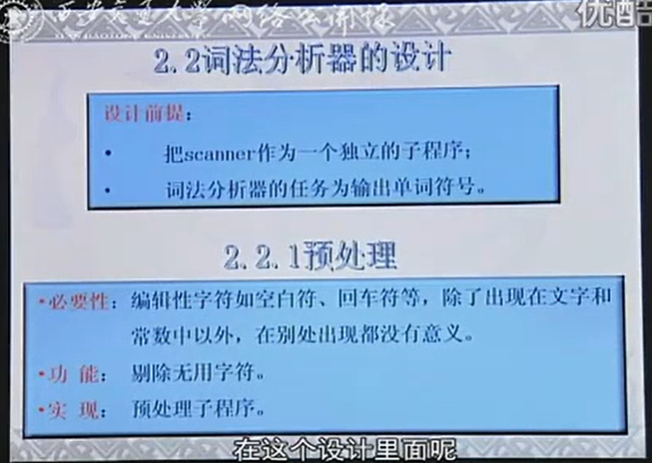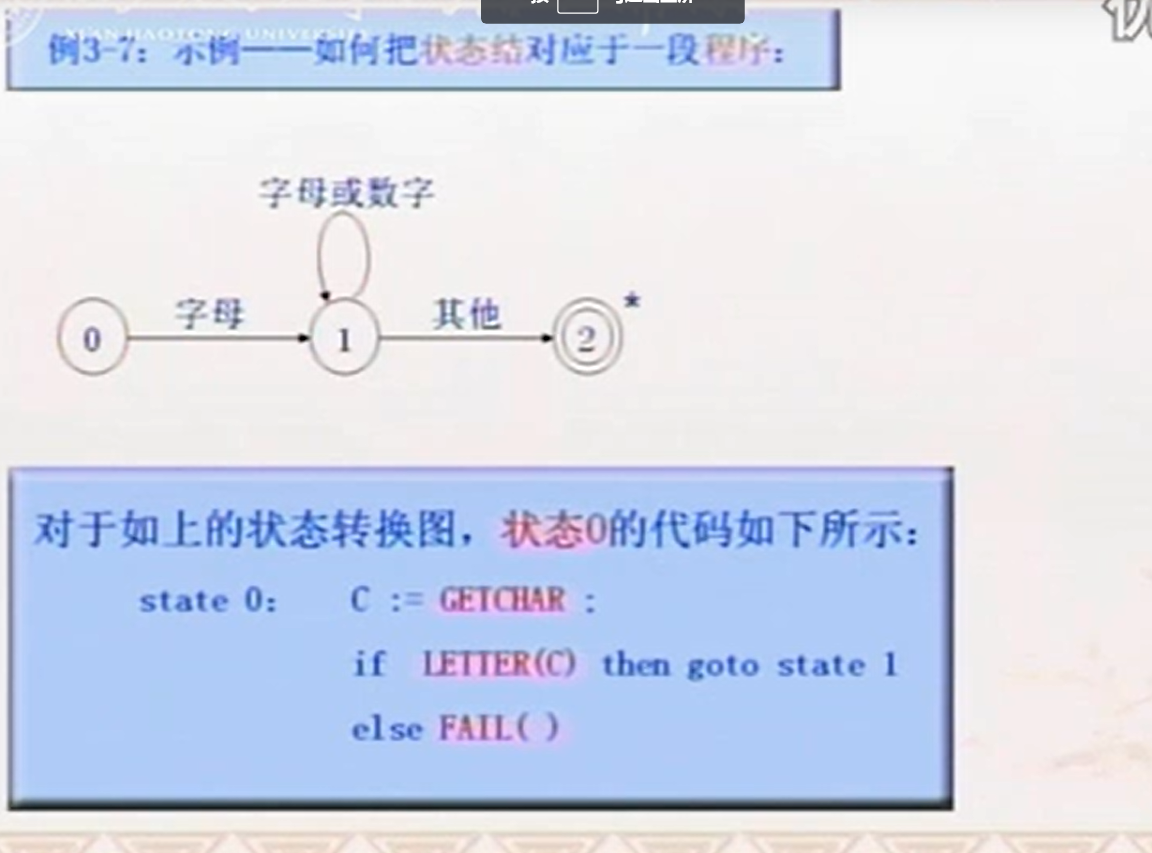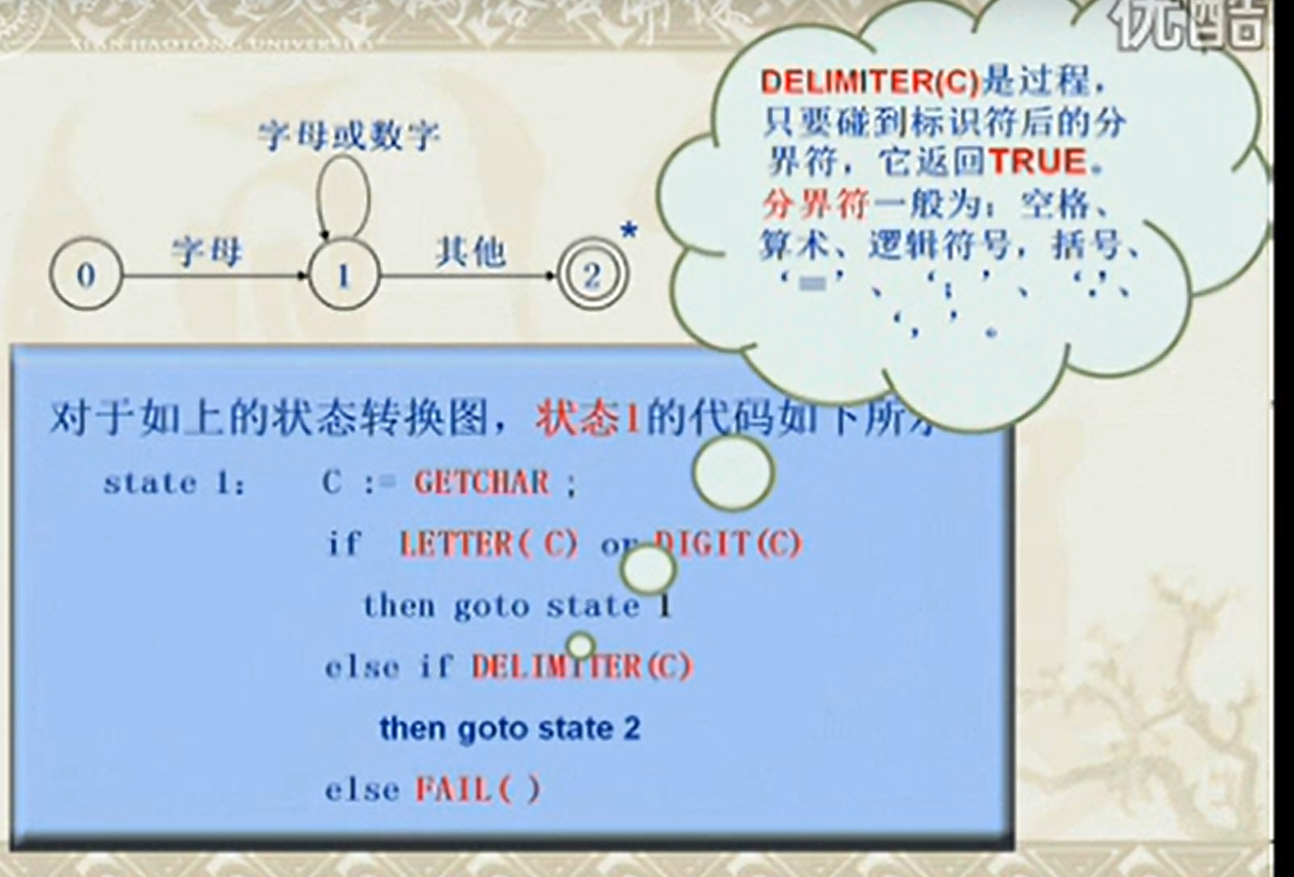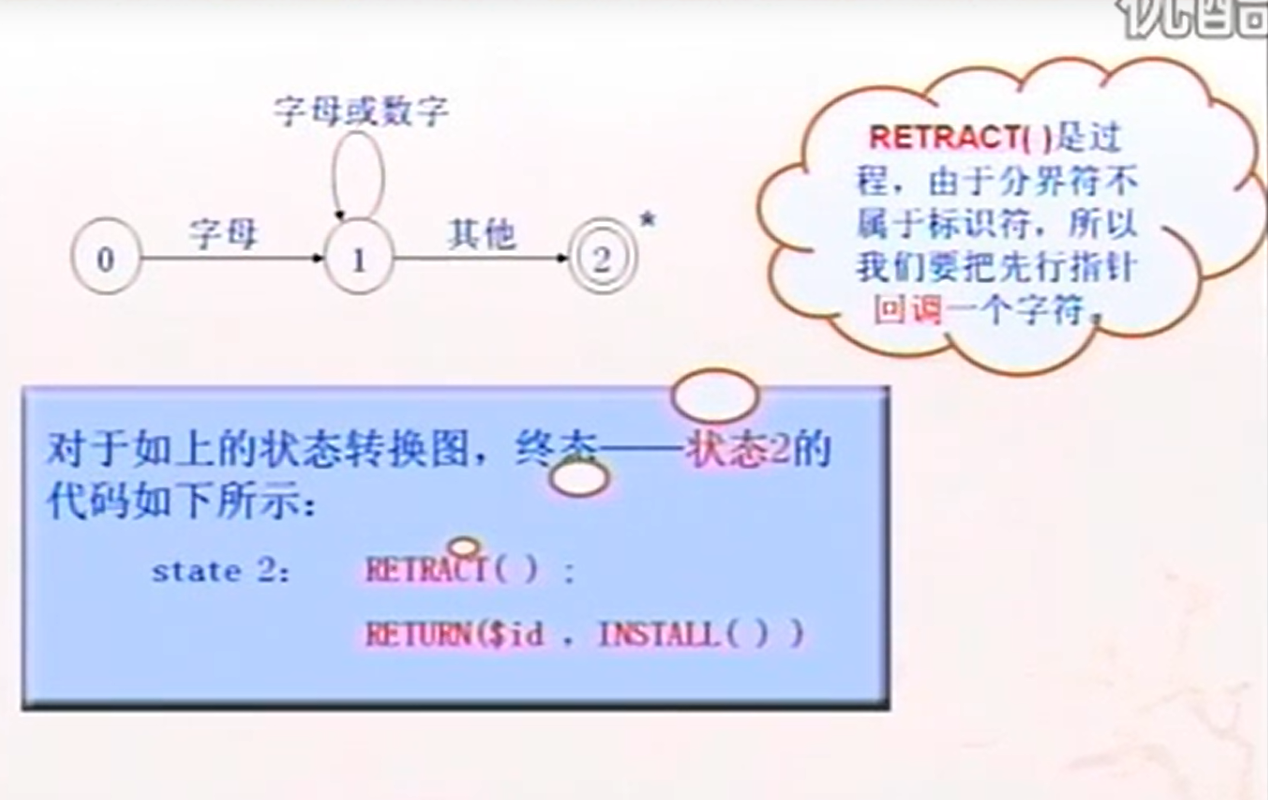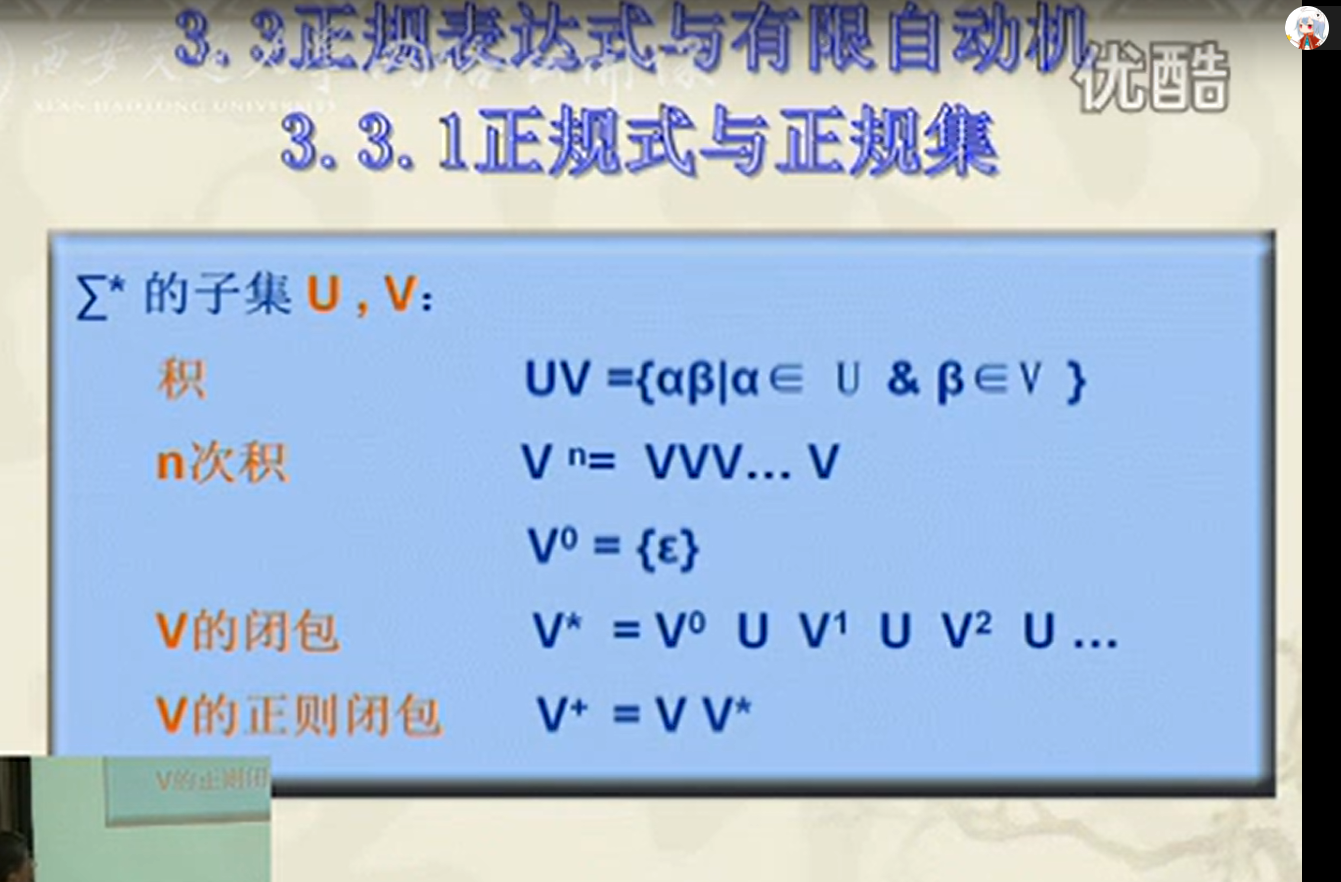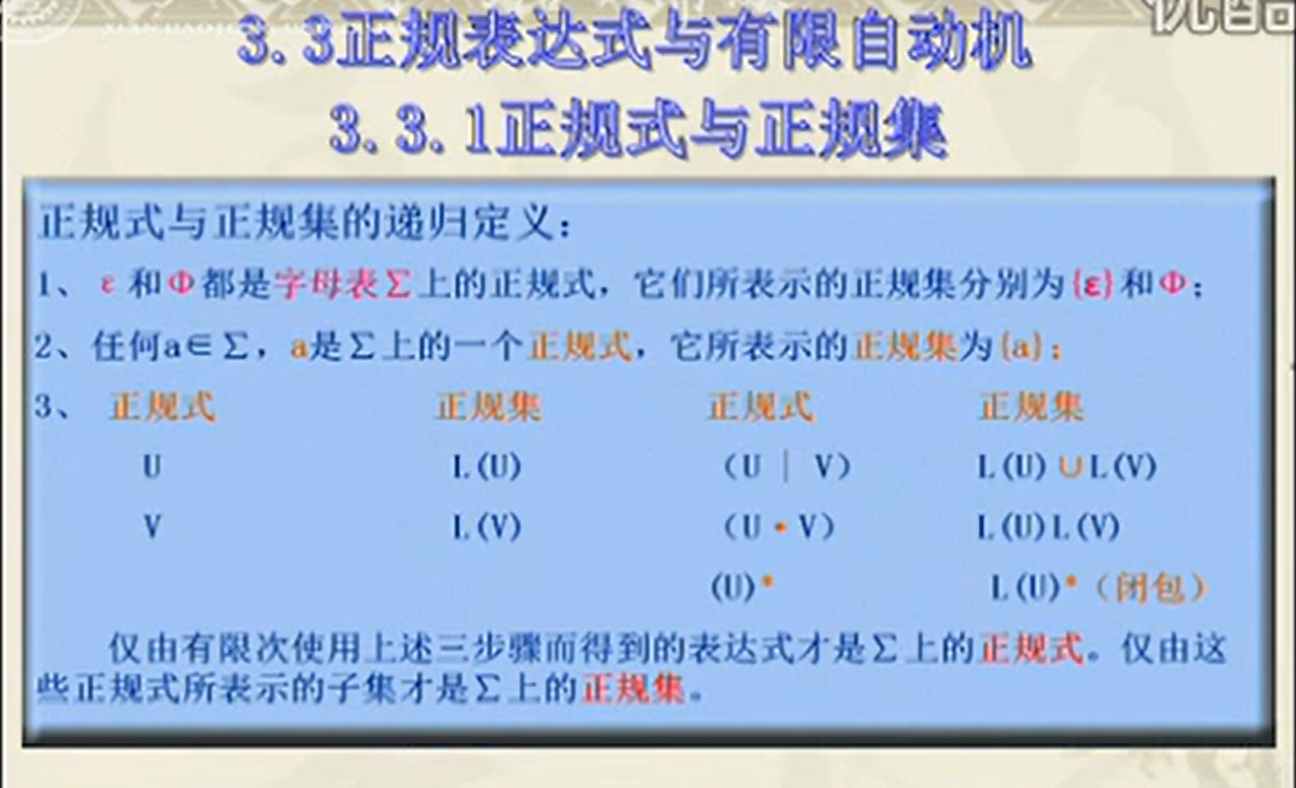展开全文• ## 编译原理词法分析

千次阅读 2016-04-20 17:45:50
编译原理词法分析词法分析的主要任务是从左至右逐个字符地对源程序进行扫描，产生一个个单词序列，用于语法分析。 1.正则表达式对给定的字符集∑={c1,c2,...,cn},归纳定义： 1.空串ε是正则表达式 2.对于任意c∈∑，...
原文地址：编译原理 词法分析编译原理

词法分析

词法分析的主要任务是从左至右逐个字符地对源程序进行扫描，产生一个个单词序列，用于语法分析。


1.正则表达式

对给定的字符集∑={c1,c2,...,cn},归纳定义：
1.空串ε是正则表达式
2.对于任意c∈∑，c是正则表达式
3.如果M和N是正则表达式，则下列表达式也是正则表达式
(1)选择 M|N={M,N}
(2)连接 MN={mn|m∈M,n∈N}
(3)闭包 M*={ε,M,MM,MMM,...}2.正则表达式的扩展

(1)[c1-cn]==c1|c2|c3|...|cn
(2)e+==一个或多个e
(3)e?==零个或一个e
(4)"a*"==a*自身，不是a的Kleen闭包
(5)e{i,j}==i到j个e的连接


3.状态转换图

状态转换图有一组被称为“状态”的结点或圆圈。词法分析器在扫描输入串的过程中寻找和某个模式匹配的词素，而
转换图中的每个状态代表一个可能在这个过程中出现的情况。


对于的状态转换图为4.有穷自动机

有穷自动机是识别器，只能对每个可能的输入串简单地回答“是”或“否”。


（1）有穷自动机分两种

1)不确定的有穷自动机（NFA）：对其边上的标号没有任何限制。一个符号标记离开同一状态的多条边，
并且空串ε也可以作为标号。
2)确定的有穷自动机（DFA）：对于每个状态及自动机输入字母表中的每个符号，有且只有一条离开该状态、以该符
号为标号的边。


（2）不确定的有穷自动机（NFA）

一个不确定的有穷自动机（NFA）由以下几个部分组成：
1)一个有穷的状态集合S。
2)一个输入符号集合∑，即输入字母表。（ε∉∑）
3)一个转换函数，它为每个状态和∑∪{ε}中的每个符号都给出了相应的后继状态的集合。
4)S中的一个状态s0被指定为开始状态，或者初始状态。
6)S的一个子集F被指定为接受状态（或终止状态）的集合。


（3）确定的有穷自动机（DFA）

确定的有穷自动机是不确定有穷自动机的一个特例。其中：
1)没有输入ε之上的转换动作。
2)对于每个状态s和每个输入符号a，有且只有一条标号为a的边离开s。


5.从正则表达式构造NFA（Thompson算法）

输入：字母表∑上的一个正则表达式r.
输出：一个接受L(r)的NFA N.
方法：首先对r进行语法分析，分解出组成它的子表达式。构造一个NFA的规则分为基本规则和归纳规则两组。
基本规则处理不包含运算符的子表达式，而归纳规则根据一个给定表达式的直接子表达式的NFA构造出这个表达式的NFA。


(1)基本规则：

1）对于表达式ε，构造下面的NFA2)对于字母表中的子表达式a，构造下面的NFA(2)归纳规则：假设正则表达式s和t的NFA分别为N(s)和N(t)

3）假设r=s|t，构造下面的NFA，这里i和f是新状态，分别是N(r)的开始状态和接受状态。从i到N(s)和N(t)的开始状态各
有一个ε转换，从N(s)和N(t)到接受状态f也各有一个ε转换。4)假设r=st，构造下面的NFA，N(s)的开始状态变成了N(r)的开始状态。N(t)的接受状态成为N(r)的唯一接受状态。N(s)的
接受状态和N(t)的开始状态合并为一个状态，合并后的状态拥有原来进入和离开合并前的两个状态的全部转换。5)假设r=s*,构造下面的NFA，i和f是两个新状态，分别是N(r)的开始状态和唯一的接受状态。要从i到达f，我们可以沿着新引入
的标号为ε的路径前进，这个路径对应于L(s)的一个串。我们也可以到达N(s)的开始状态，然后经过该NFA，再零次或多次从它的
接受状态回到它的开始状态并重复上述过程。(3)利用Thompson算法为正则表达式r=(a|b)*abb构造一个NFA

1)首先画出语法分析树2)对于表达式r1=a，r2=b构造NFA3)对于表达式r3=r1|r2构造NFA4)对于表达式r5=r3*构造NFA5)对于表达式r6=r1r2构造NFA6)对于表达式r7=r5r6构造NFA7)同理最后可以得到NFA6.由NFA构造DFA的子集构造算法（subset construction）

输入：一个NFA N.
输出：一个接受同样语言的DFA D.
方法：我们为D构造一个转换表Dtran，D的每个状态是一个NFA状态集合，我们将构造Dtran，使得D"并行地"模拟N在遇到一个
给定输入串时可能执行的所有动作。我们面对的第一个问题是正确处理N的ε转换。


下面给定了一些函数的定义，这些函数描述了一些需要在这个算法中执行的N的状态集上的基本操作，s表示N的单个状态，T代表N的一个状态集。我们需要找出当N读入了某个输入串之后可能位于的所有状态集合。
首先，在读入第一个输入符号之前，N可以位于集合ε-closure(s0)中的任何状态上，其中s0是N的开始状态。下面进行归纳定义，
假定N在读入输入串x之后可以位于集合T中的状态上。如果下一个输入符号是a，那么N可以立即移动到move(T,a)中的任何状态。
然而，N可以在读入a后再执行几个ε转换，因此N在读入xa之后可位于ε-closure(move(T,a))中的任何状态上。ε-closure(T)的计算：从一个状态集合开始，所有只存在标号为ε的边都加入T对于上面由Thompson算法为正则表达式r=(a|b)*abb构造一个NFA，我们将这个NFA转换成一个DFA首先，NFA的开始状态A是ε-closure(0)，即A={0，1，2，4，7}，NFA的输入字母表是{a,b}，则
Dtran[A,a]=ε-closure(move(A,a))=ε-closure({3,8})={1,2,3,4,6,7,8},令B=Dtran[A,a]
Dtran[A,b]=ε-closure(move(A,b))=ε-closure({5})={1,2,4,6,7},令C=Dtran[A,b]
Dtran[B,a]=ε-closure(move(B,a))=ε-closure({3,8})={1,2,3,4,6,7,8}=B
Dtran[B,b]=ε-closure(move(B,b))=ε-closure({5,9})={1,2,4,5,6,7,9},令D=Dtran[B,b]
Dtran[C,a]=ε-closure(move(C,a))=ε-closure({3,8})={1,2,3,4,6,7,8}=B
Dtran[C,b]=ε-closure(move(C,b))=ε-closure({5})={1,2,4,6,7}=C
Dtran[D,a]=ε-closure(move(D,a))=ε-closure({3,8})={1,2,3,4,6,7,8}=B
Dtran[D,b]=ε-closure(move(D,b))=ε-closure({5,10})={1,2,4,5,6,7,10},令E=Dtran[D,b]
Dtran[E,a]=ε-closure(move(E,a))=ε-closure({3,8})={1,2,3,4,6,7,8}=B
Dtran[E,b]=ε-closure(move(E,b))=ε-closure({5})={1,2,4,6,7}=C


所有DFA的转换表Dtran根据转换表可以得到DFA7.最小化一个DFA的状态数量

输入：一个DFA D，其状态集合为S，输入字母表为∑，开始状态为s0，接受状态为F。
输出：一个DFA D'，它和D接受同样的语言，且状态数最少。
方法：
1)首先构造包含两个组F和S-F的初始划分II，这两个组分别是D的接受状态组和非接受状态组。
2)用下面的构造新的分划IInew3)如果IInew=II,令IIfinal=II并接着执行步骤4)，否则，用IInew替换II并重复步骤2)。
4)在分划IIfinal的每个组中选取一个状态作为该组的代表。这些代表构成了状态最少DFA D'的状态。
D'的其他部分按如下步骤构建：
(a)D'的开始状态是包含了D的开始状态的组的代表。
(b)D'的接受状态是那些包含了D的接受状态的组的代表。
(c)令s是IIfinal中某个组G的代表，并令DFA D中在输入a上离开s的转换到达状态t。令r为t所在组H的代表。
那么在D'中存在一个从s到r在输入a上的转换。


对上面的DFA最小化首先初始划分包括两个组{A,B,C,D},{E},分别是非接受状态组和接受状态组。构造IInew时，考虑这两个组
和输入符号a和b，因为组{E}只包含一个状态，不能再被分割，所以{E}被原封不动的保留在IInew中。对于
{A,B,C,D}，是可以被分割的，因此我们必须考虑各个输入符号的作用，在输入a上，这些状态中的每一个都
转到B，因此使用以a开头的串无法区分这些状态。但对于输入b，状态A、B、C都转换到{A,B,C,D}的某个成
员上，而D转到另一组中的成员E上，因此在IInew中，{A,B,C,D}被分割成{A,B,C}，{D}，现在IInew中有
{A,B,C}，{D}，{E}。对于{A,B,C}，在输入b上，A和C都到达{A,B,C}中的元素，B却到达D上，所有IInew
有{A,C},{B},{D},{E},对于{A,C}无法在分割。
所有最后有{A,C},{B},{D},{E}，构造如下的DFA展开全文正则表达式 闭包
• 编译原理词法分析器编译原理是个很难的学科，老师这么说的，第一个实验就是写一个简单的词法分析器。分析器的作用就是能够从文本中分离出相应的保留字、标识符、界符、数字等等。本例具体要求如下：1、根据以下的...c++
• 2 用flex词法分析生成器进行词法分析 五、实验结果 1 直接扫描法 2 flex词法分析生成器进行词法分析 参考资料 附录 1 直接扫描法代码 2 hide-digits.l文件 一、实验目的 1、掌握直接扫描法； 2、了解...python 正则表达式 字符串
• 深刻领会状态转换图的含义，逐步理解有限自动机。 掌握手工生成词法分析器的方法，了解词法分析器的内部工作原理。 实验内容 (自己设计的某种计算机语言)的编译程序的词法分析部分实现。 从左到右扫描...
• 例如，可根据文法或状态转换图构造相应的状态矩阵，该状态矩阵同控制程序便组成了编译程序的词法分析程序；也可以根据文法或状态转换图利用某种语言（汇编语言或高级语言）直接编写词法分析程序。
• 编译原理实验报告 计算机与信息学院 第 第 PAGE 14 页 共 NUMPAGES 14 页 实验一 词法分析器的设计 一实验目的 理解词法分析器的任务和输出形式 理解扫描器的工作原理 掌握状态转换图的绘制以及单词的识别技术 掌握...
• 上一篇文章我们介绍了在词法分析中涉及到的词法单元、模式和词素的概念，并给出了正则表达式的递归定义，以及如何把一个正则表达式转换成一个状态转换图。本篇文章将接着上一篇文章的内容，继续介绍词法分析的一个...
• 1.根据状态转换图直接编程的方式；2.利用DFA编写通用的词法分析程序。 二、实验内容及要求 1．根据保留字和特殊符号表能区分出源文件中的保留字、普通标识符和特殊符号，并能进行简单的错误处理。 2．设计词法分析器...
• 算法：使用状态转换图实现 程序代码： /* 关键字：<AUTO, >；标示符：<IDENT,my_name>；整型常量<200,数值>；实型常量<300,数值>；分隔符：<400-408, >；运算符：<500-, > */ ...C++
• 词法分析（英语：lexical analysis）是计算机科学中将字符序列转换为单词（Token）序列的过程。进行词法分析的程序或者函数叫作词法分析器（Lexical analyzer，简称Lexer），也叫扫描器（Scanner）。词法分析器一般...java
• 将正则表达式表示的模式构造为状态转换图. 在本文中只列举状态转换图. 双缓冲区(代码中的Buffer类) 数字的状态转换 保留字和ID的状态转换 运算符的状态转换 用于分析的源文件 结果 前情提要 一、词素模式 二、...c++
• 通过正则表达式实现对单词的识别项目描述：通过状态转换图实现对单词的识别 - 输入：符号串输出：yes/no
• 转发自： 作者：jzyhywxz  ... 本文是词法分析的第一篇文章，主要介绍在词法分析过程中需要用到的一些基本概念，包括词法单元、模式和词素以及三者之间的关系，理解这些内容对学习词法分析过程十分重要。 ...
• 本文首先将介绍如何把一个正则表达式转换成一个有穷自动机，接着会给出一个最小化DFA状态数的算法，最后会回顾整个词法分析过程。 从正则表达式到有穷自动机 对我们来说，用正则表达式描述一种语言是十分方便的。...
• 举例：试证baab可被下面的DFA所接受。 DFA的确定性表现在转换函数f: K×Σ→K是一个...从状态转换图来看，若字母表含有n个输入符号，那么任何一个状态结点最多有n条弧射出，而且每条弧以一个不同的输入符号进行标记。
• 要求：阐述词法分析系统所要完成的功能 词法分析程序的主要任务是将源程序根据单词进行切分，输出相应的token序列。具体地，本程序完成的功能如下： 基本功能： 能够识别以下内容： 1.标识符：（由大小写字母、数字...java...